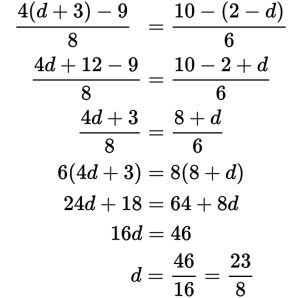# SAT Math Multiple Choice Question 305: Answer and Explanation

### Test Information

Question: 305

5.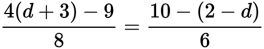In the equation above, what is the value of d?

• A.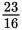• B.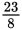• C.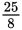• D.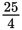Explanation:

B

Difficulty: Medium

Category: Heart of Algebra / Linear Equations

Strategic Advice: Choose the best strategy to answer the question. You could start by cross-multiplying to get rid of the denominators, but simplifying the numerators first will make the calculations easier.